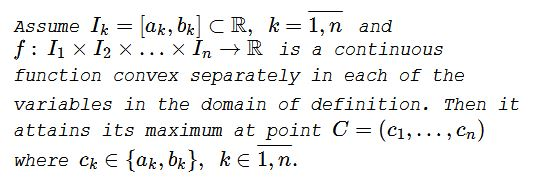# Gireaux's Theorem

### Theorem

If a continuous function of several variables is defined on a hyperbrick and is convex in each of the variables, it attains its maximum at one of the corners. More formally:The statement of the theorem is a specification of a theorem of Weierstrass (the Extreme Values Theorem) that states that a continuous function defined on a compact set attains its extremes in the set. Assume now that the function is convex in each of its variables (i.e., as a function of one argument, with other arguments fixed.) A continuous function of one variable, convex on a closed interval, attains its maximum at one of the endpoints of the interval. This means that the maximum of the given function is attained at either, say, ${a_1}\times I_2\times\ldots\times I_n\,$ or ${b_1}\times I_2\times\ldots\times I_n,\,$ which reduces the dimension of the search for the maximum by $1.\,$ Doing this recursively proves the statement.

References

1. Israel Meireles Chrisostomo, Trigonometria Pura e Aplicações e um pouco além: problemas de Olimpíadas, 3 de Julho, 2015

### USA 1980

Prove that, for $a,b,c\in[0,1],$

$\displaystyle\small{\frac{a}{b+c+1}+\frac{b}{c+a+1}+\frac{c}{a+b+1}+(1-a)(1-b)(1-c)\le 1}.$

The function $f(a,b,c)=\displaystyle \sum_{cycl}\frac{a}{b+c+1}+\prod_{cycl}(1-a)\,$ is convex in each of the three variables $a,b,c,\,$ so that $f\,$ takes its maximum value in one of either vertices of the cube $0\le a\le 1,\,0\le b\le 1,\,0\le c\le 1.\,$ Since $f(a,b,c)\,$ takes value $1\,$ in each of these points, the required inequality is proven.

References

1. M. S. Klamkin, USA Mathematical Olympiads 1972-1986, MAA, 1988

### Dan Sitaru I

Prove that, for $a,b,c,d\in [0,2],$

$\displaystyle\small{\frac{9a}{1+bcd}+\frac{9b}{1+cda}+\frac{9c}{1+dab}+\frac{9d}{1+abc}+9e^{abcd}\leq 8+9e^{16}}.$

$f:\,[0,2]^4\to \mathbb{R},\,$ $\displaystyle f(a,b,c,d)=9\sum \frac{a}{1+bcd}+9e^{abcd}.\,$

$\displaystyle f'_a=\frac{9}{1+bcd}-\frac{9bcd}{(1+cda)^2}-\frac{9cdb}{(1+dab)^2}-\frac{9dbc}{(1+abc)^2}+9bcde^{abcd},$

$\displaystyle f''_{aa}=\frac{18bc^2d^2}{(1+cda)^3}+\frac{18cd^2b^2}{(1+dab)^3}+\frac{18db^2c^2}{(1+abc)^3}+9b^2c^2d^2 e^{abcd}\gt 0.$

$f\,$ strictly convex in variable $a\,$ and, similarly, in the rest of the variables. $f\,$ defined on a compact set $[0,2]^4,\,$ hence, by Gireaux's theorem $f\,$ attains it maximum at the vertices of the hypercube $[0,1]^4.\,$ It is easy to check that the maximum is attained for $f(2,2,2,2)=4\cdot \frac{18}{1+8}+9e^{16}=8+9e^{16},\,$ thus proving the inequality.

### Dan Sitaru II

Prove that, for $x,y,z\in [0,1],$

$\displaystyle\small{\frac{x}{y+z+2016}+\frac{y^2}{z+x+2016}+\frac{z^3}{x+y+2016}+(1-x)(1-y)(1-z)\le 1}.$

$f:\,[0,2]^3\to \mathbb{R},$

$\displaystyle f(x,y,z)=\frac{x}{y+z+2016}+\frac{y^2}{z+x+2016}+\frac{z^3}{x+y+2016}\\ \qquad\qquad+(1-x)(1-y)(1-z)$

We easily check that

$\displaystyle f'_xx=\frac{2y^2}{(x+z+2016)^3}+\frac{2z^3}{(x+y+2016)^3}\gt 0.$

$f\,$ strictly convex in variable $a\,$ and, similarly, in the rest of the variables. $f\,$ defined on a compact set $[0,2]^4,\,$ hence, by Gireaux's theorem $f\,$ attains it maximum at the vertices of the hypercube $[0,1]^3.\,$ It is easy to check that the maximum is attained for $f(0,0,0)=1,\,$ thus proving the inequality.

Second proof (Leo Guigiuc)

From $x,y,z\in [0,1]\,$ it follows that $\displaystyle\frac{x}{y+z+2016}\le\frac{x}{3};\,$ $\displaystyle \frac{y^2}{z+x+2016}\le\frac{y}{3},\,$ $\displaystyle\frac{z^3}{x+y+2016}\le\frac{z}{3}.\,$ Thus suffice it to prove that

$\displaystyle f(x,y,z)=\frac{x+y+z}{3}+(1-x)(1-y)(1-z)\le 1.$

Let $a=1-x,\,$ $b=1-y,\,$ $c=1-z.\,$ The inequality to prove becomes

$\displaystyle \frac{1-a+1-b+1-c}{3}+abc\le 1,$

or, $\displaystyle abc\le\frac{a+b+c}{3},\,$ which is true because, for $a,b,c\in [0,1],\,$ $abc\le\sqrt{abc}\,$ and by the AM-GM inequality.

Third proof

Observe that $f(x,y,z)\,$ defined in the second proof is linear, hence convex, in each of its arguments. Gireaux's theorem applies. $f(0,0,0)=1,\,$ $f(0,0,1)=\displaystyle\frac{1}{3},\,$ $f(0,1,1)=\displaystyle\frac{2}{3},\,$ $f(1,1,1)=\displaystyle\frac{1}{3}=1.$

### Acknowledgment

I am indebted to Dan Sitaru for supplying the references and the examples.